Скачать презентацию Bond Valuation Investors Buyers Bond Contract Issuer

ac4f82e0c5eb5373307807b1890383fe.ppt

• Количество слайдов: 18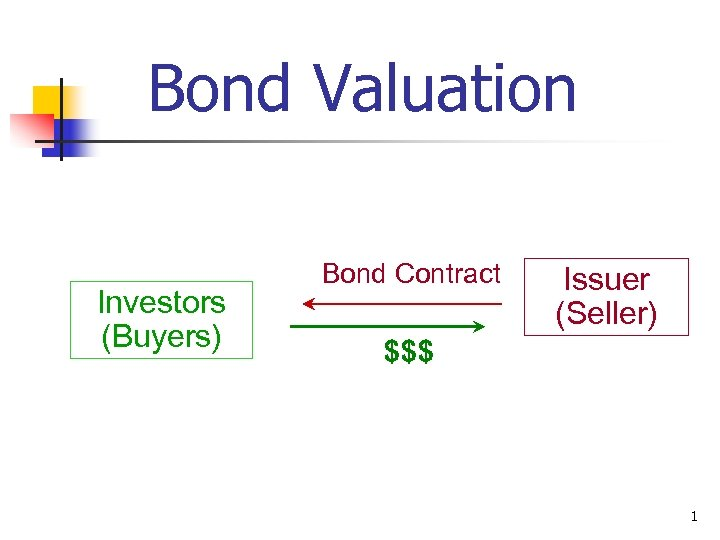Bond Valuation Investors (Buyers) Bond Contract \$\$\$ Issuer (Seller) 1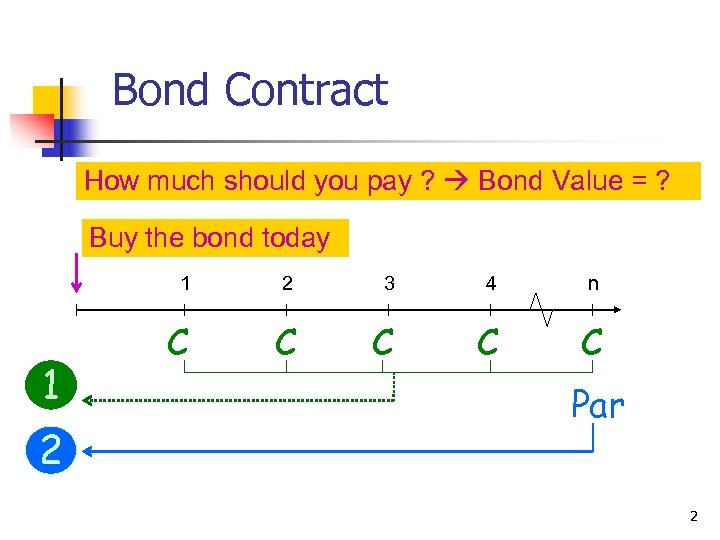Bond Contract How much should you pay ? Bond Value = ? Buy the bond today 1 1 2 2 3 4 n C C C Par 2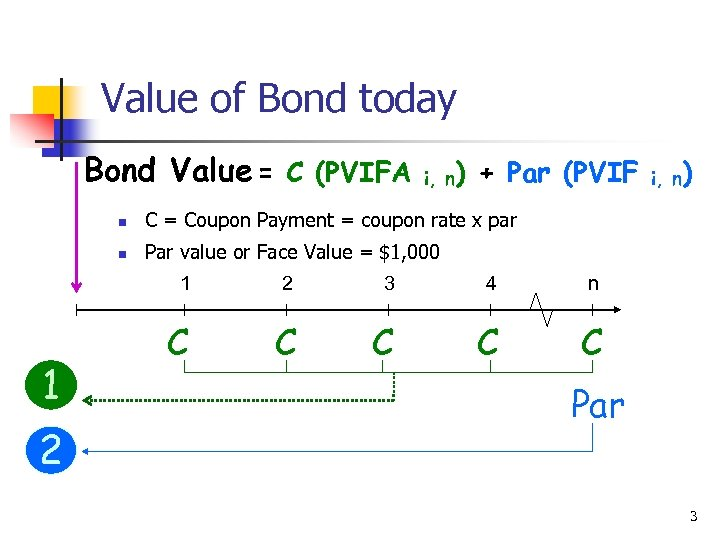Value of Bond today Bond Value = C (PVIFA i, n) + Par (PVIF n C = Coupon Payment = coupon rate x par n Par value or Face Value = \$1, 000 1 1 2 i, n) 2 3 4 n C C C Par 3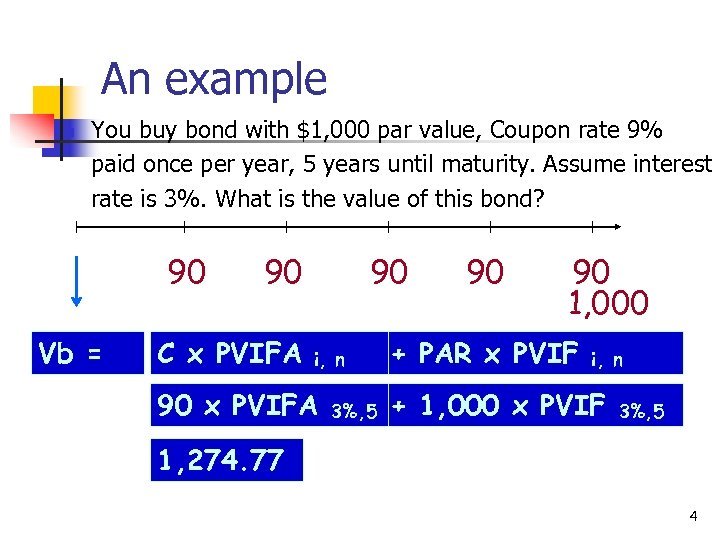An example n You buy bond with \$1, 000 par value, Coupon rate 9% paid once per year, 5 years until maturity. Assume interest rate is 3%. What is the value of this bond? 90 Vb = 90 C x PVIFA 90 i, n 90 x PVIFA 3%, 5 90 90 1, 000 + PAR x PVIF i, n + 1, 000 x PVIF 3%, 5 1, 274. 77 4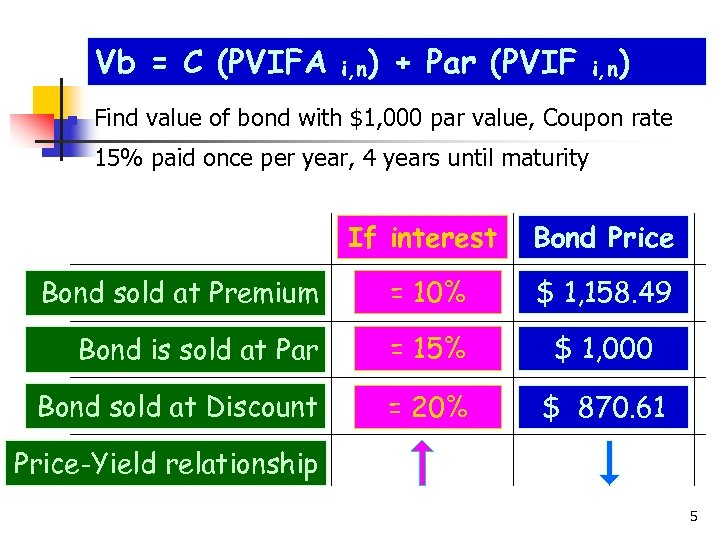Vb = C (PVIFA n i, n) + Par (PVIF i, n) Find value of bond with \$1, 000 par value, Coupon rate 15% paid once per year, 4 years until maturity If interest Bond Price Bond sold at Premium = 10% \$ 1, 158. 49 Bond is sold at Par = 15% \$ 1, 000 Bond sold at Discount = 20% \$ 870. 61 Price-Yield relationship 5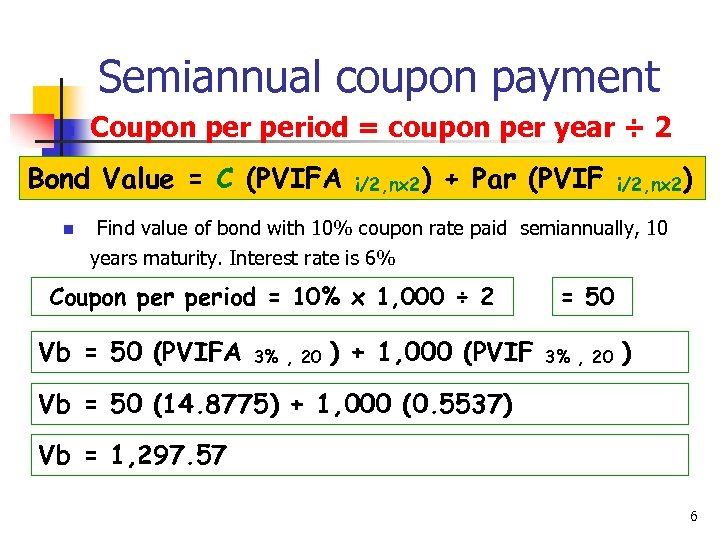Semiannual coupon payment n Coupon period = coupon per year ÷ 2 Bond Value = C (PVIFA n i/2, nx 2) + Par (PVIF i/2, nx 2) Find value of bond with 10% coupon rate paid semiannually, 10 years maturity. Interest rate is 6% Coupon period = 10% x 1, 000 ÷ 2 Vb = 50 (PVIFA 3% , 20 ) + 1, 000 (PVIF = 50 3% , 20 ) Vb = 50 (14. 8775) + 1, 000 (0. 5537) Vb = 1, 297. 57 6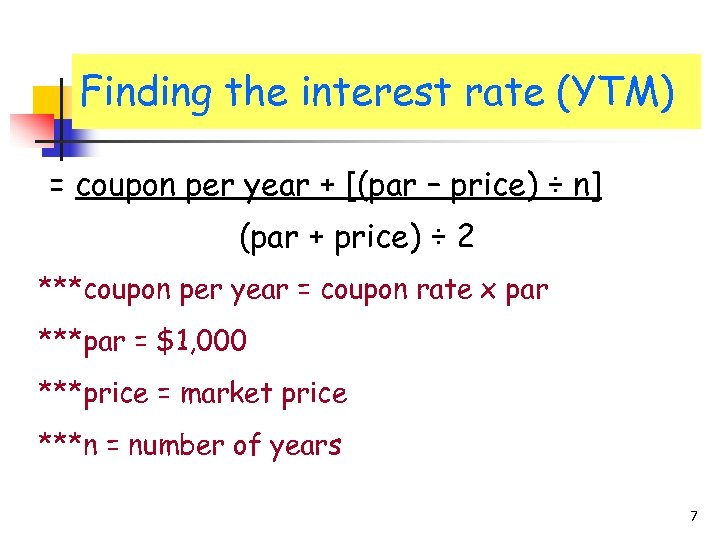Finding the interest rate (YTM) = coupon per year + [(par – price) ÷ n] (par + price) ÷ 2 ***coupon per year = coupon rate x par ***par = \$1, 000 ***price = market price ***n = number of years 7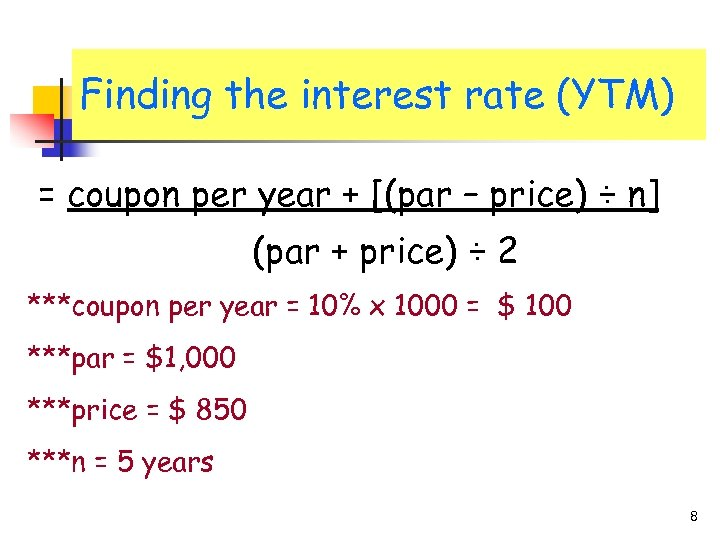Finding the interest rate (YTM) = coupon per year + [(par – price) ÷ n] (par + price) ÷ 2 ***coupon per year = 10% x 1000 = \$ 100 ***par = \$1, 000 ***price = \$ 850 ***n = 5 years 8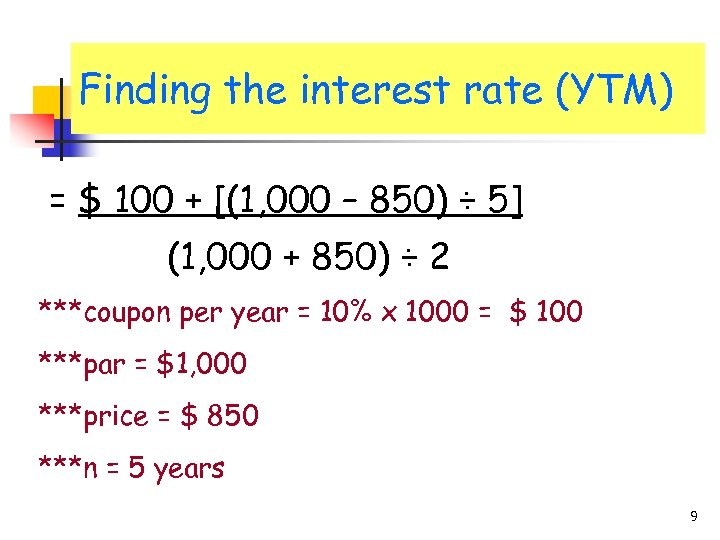Finding the interest rate (YTM) = \$ 100 + [(1, 000 – 850) ÷ 5] (1, 000 + 850) ÷ 2 ***coupon per year = 10% x 1000 = \$ 100 ***par = \$1, 000 ***price = \$ 850 ***n = 5 years 9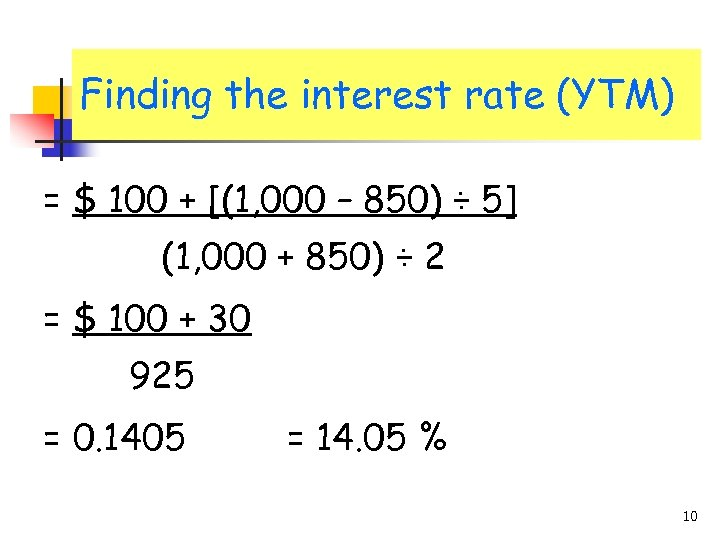Finding the interest rate (YTM) = \$ 100 + [(1, 000 – 850) ÷ 5] (1, 000 + 850) ÷ 2 = \$ 100 + 30 925 = 0. 1405 = 14. 05 % 10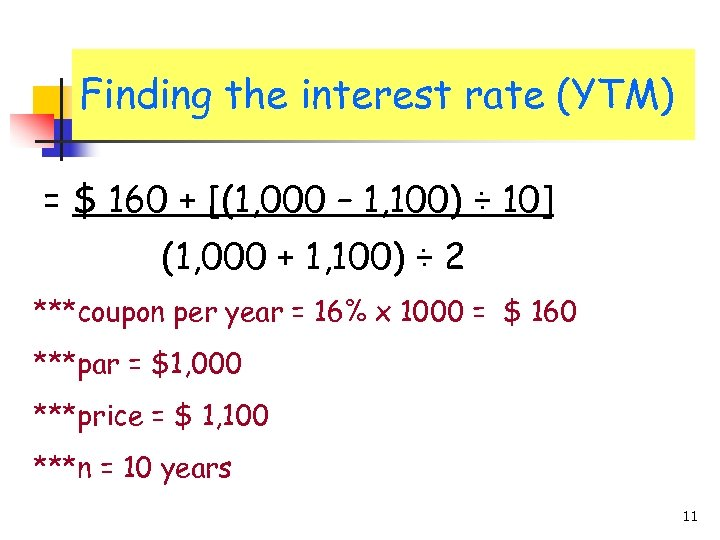Finding the interest rate (YTM) = \$ 160 + [(1, 000 – 1, 100) ÷ 10] (1, 000 + 1, 100) ÷ 2 ***coupon per year = 16% x 1000 = \$ 160 ***par = \$1, 000 ***price = \$ 1, 100 ***n = 10 years 11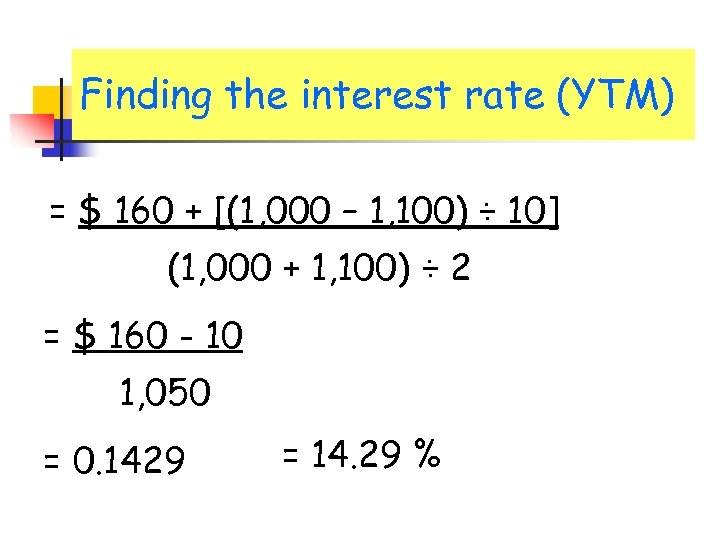Finding the interest rate (YTM) = \$ 160 + [(1, 000 – 1, 100) ÷ 10] (1, 000 + 1, 100) ÷ 2 = \$ 160 - 10 1, 050 = 0. 1429 = 14. 29 %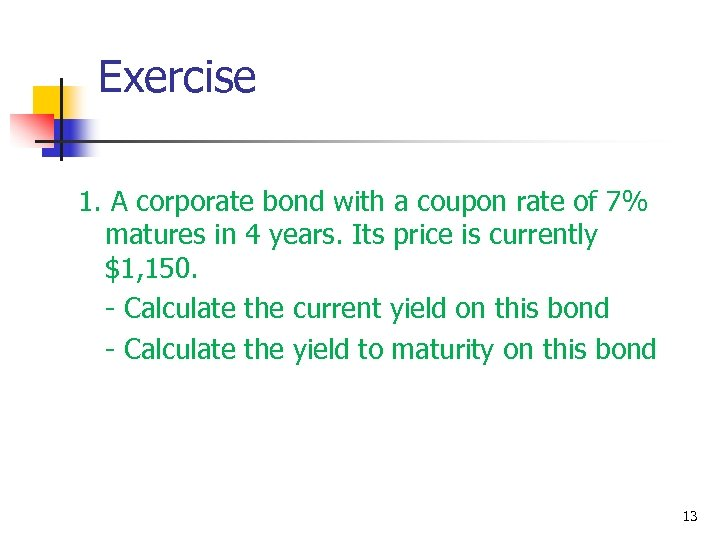Exercise 1. A corporate bond with a coupon rate of 7% matures in 4 years. Its price is currently \$1, 150. - Calculate the current yield on this bond - Calculate the yield to maturity on this bond 13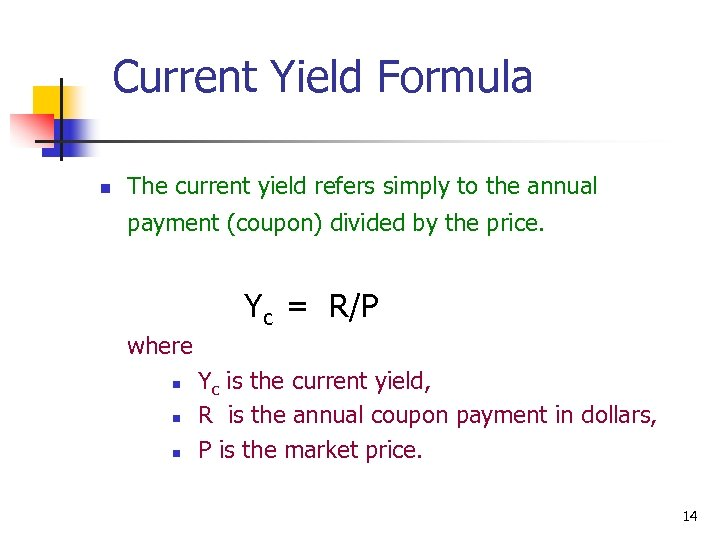Current Yield Formula n The current yield refers simply to the annual payment (coupon) divided by the price. Yc = R/P where n n n Yc is the current yield, R is the annual coupon payment in dollars, P is the market price. 14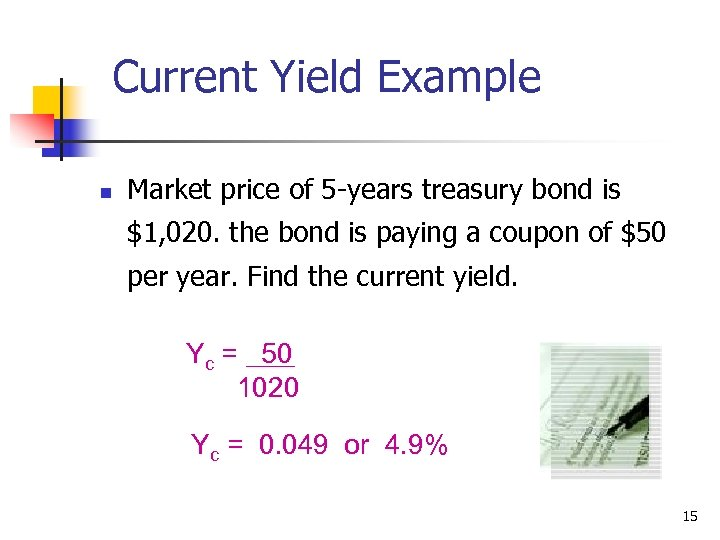Current Yield Example n Market price of 5 -years treasury bond is \$1, 020. the bond is paying a coupon of \$50 per year. Find the current yield. Yc = 50 1020 Yc = 0. 049 or 4. 9% 15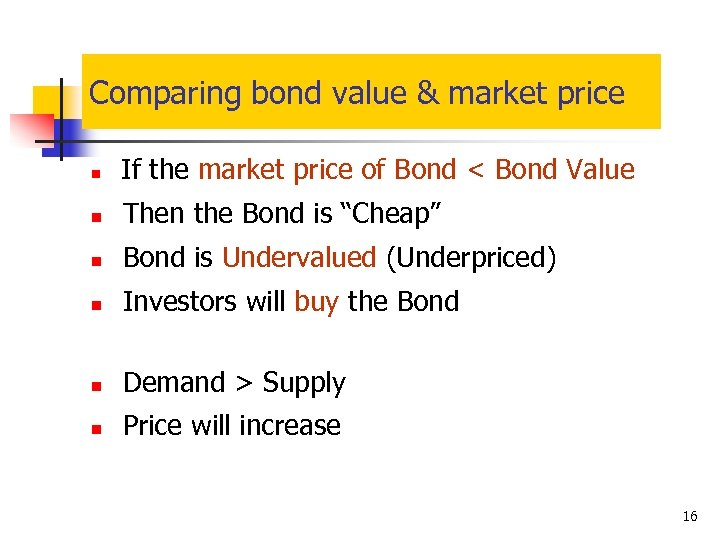Comparing bond value & market price n If the market price of Bond < Bond Value n Then the Bond is “Cheap” n Bond is Undervalued (Underpriced) n Investors will buy the Bond n Demand > Supply n Price will increase 16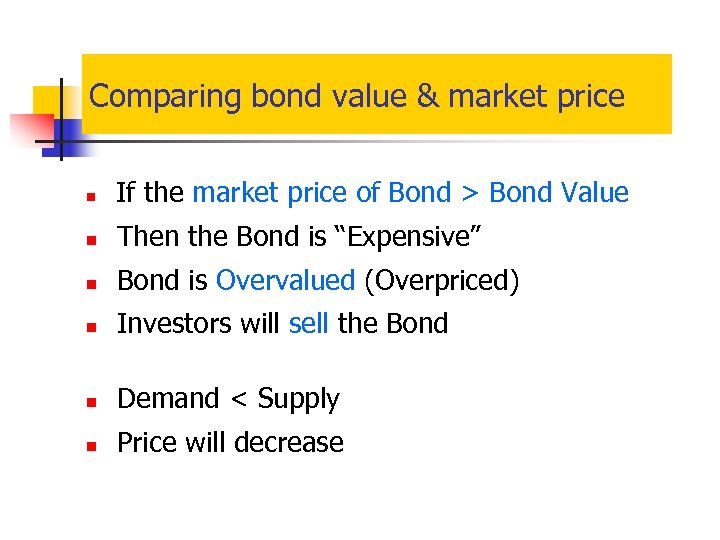Comparing bond value & market price n If the market price of Bond > Bond Value n Then the Bond is “Expensive” n Bond is Overvalued (Overpriced) n Investors will sell the Bond n Demand < Supply n Price will decrease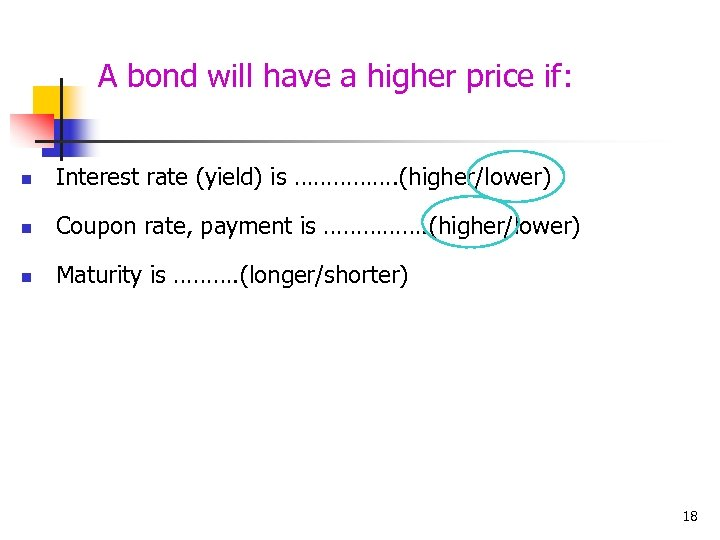A bond will have a higher price if: n Interest rate (yield) is ……………. (higher/lower) n Coupon rate, payment is ……………. (higher/lower) n Maturity is ………. (longer/shorter) 18